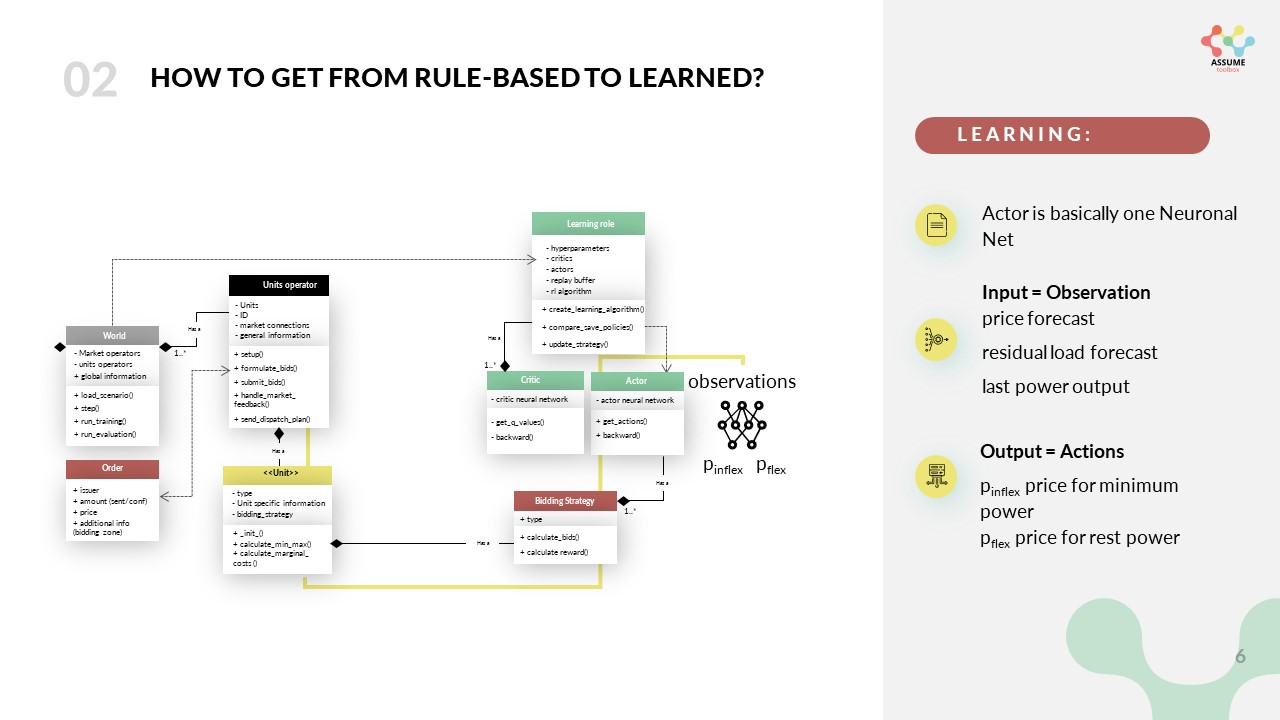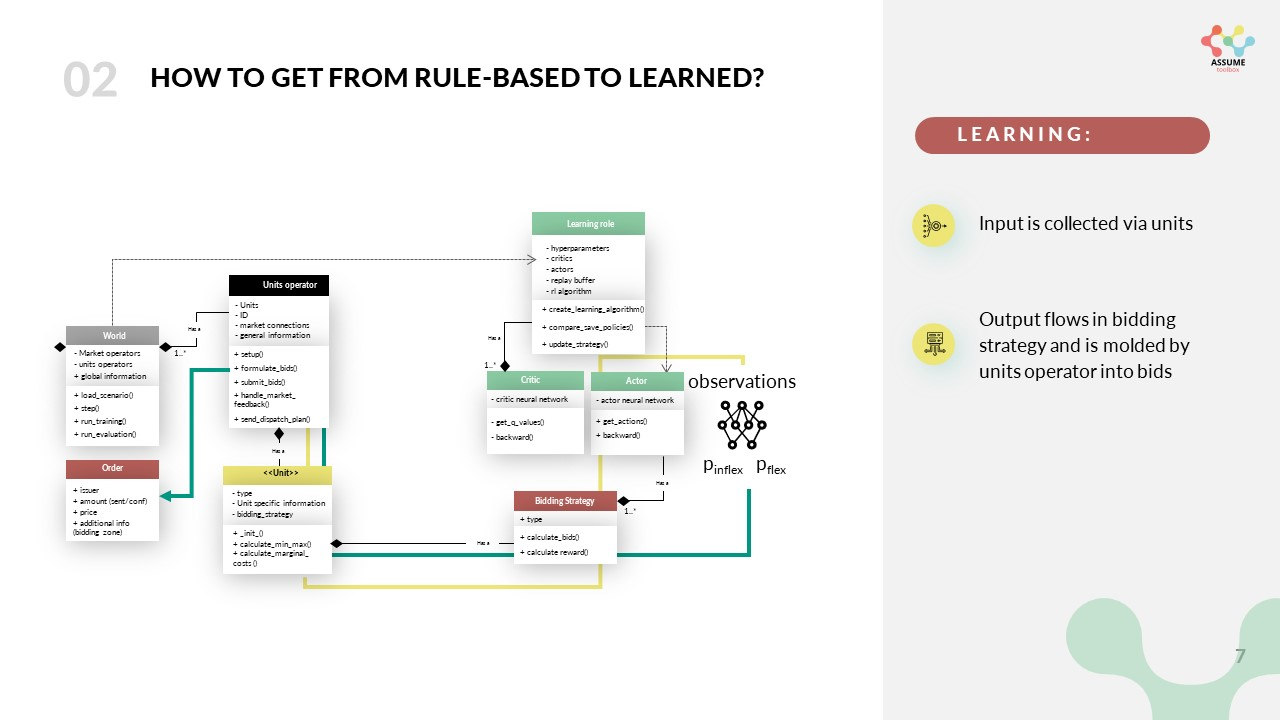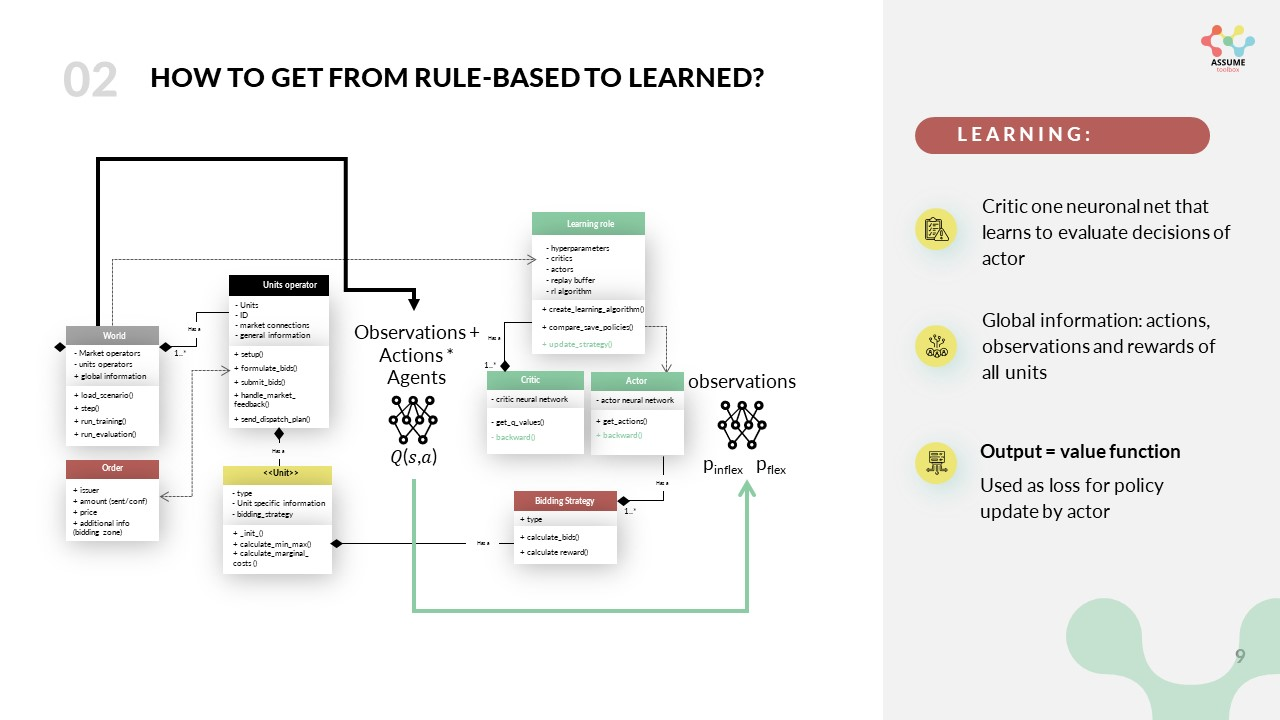# Reinforcement Learning#

One unique characteristic of ASSUME is the usage of Reinforcement Learning (RL) for the bidding of the agents. To enable this the architecture of the simulation is designed in a way to accommodate the learning process. In this part of the documentation, we give a short introduction to reinforcement learning in general and then pinpoint you to the relevant parts of the code. If you want a hands-on introduction check out the prepared tutorial in Colab: https://colab.research.google.com/drive/1LISiM1QvDIMXU68pJH-NqrMw5w7Awb24?usp=sharing

## The Basics of Reinforcement Learning#

In general RL and deep reinforcement learning (DRL), in particular, open new prospects for agent-based electricity market modeling. Such algorithms offer the potential for agents to learn bidding strategies in the interplay between market participants. In contrast to traditional rule-based approaches, DRL allows for a faster adaptation of the bidding strategies to a changing market environment, which is impossible with fixed strategies that a market modeller explicitly formulates. Hence, DRL algorithms offer the potential for simulated electricity market agents to develop bidding strategies for future markets and test emerging markets’ mechanisms before their introduction into real-world systems.

In the current implementation of ASSUME, we model the electricity market as a partially observable Markov game, which is an extension of MDPs for multi-agent setups. Multi-agent DRL is understood as the simultaneous learning of multiple agents interacting in the same environment. The Markov game for $$N$$ agents consists of a set of states $$S$$, a set of actions $$A_1, \ldots, A_N$$, a set of observations $$O_1, \ldots, O_N$$, and a state transition function $$P: S \times A_1 \times \ldots \times A_N \rightarrow \mathcal{P}(S)$$ dependent on the state and actions of all agents. After taking action $$a_i \in A_i$$ in state $$s_i \in S$$ according to a policy $$\pi_i: O_i \rightarrow A_i$$, every agent $$i$$ is transitioned into the new state $$s'_i \in S$$. Each agent receives a reward $$r_i$$ according to the individual reward function $$R_i$$ and a private observation correlated with the state $$o_i: S \rightarrow O_i$$. Like MDP, each agent $$i$$ learns an optimal policy $$\pi_i^*(s)$$ that maximizes its expected reward.

To enable multi-agent learning some adjustments are needed within the learning algorithm to get from the TD3 to an MATD3 algorithm. Other authors used similar tweaks to improve the MADDPG algorithm and derive the MA-TD3 algorithm. We’ll start explaining the learning by focusing on a single agent and then extend it to multi-agent learning.

### Single-Agent Learning#

We use the actor-critic approach to train the learning agent. The actor-critic approach is a popular RL algorithm that uses two neural networks: an actor network and a critic network. The actor network is responsible for selecting actions, while the critic network evaluates the quality of the actions taken by the actor.

#### Actor#

The actor network is trained using the policy gradient method, which updates the weights of the actor network in the direction of the gradient of the expected reward with respect to the network parameters:

$\nabla_{\theta} J(\theta) = E[\nabla_{\theta} log \pi_{\theta}(a_t|s_t) * Q^{\pi}(s_t, a_t)]$

where $$J(\theta)$$ is the expected reward, $$\theta$$ are the weights of the actor network, $$\pi_{\theta}(a_t|s_t)$$ is the probability of selecting action $$a_t$$ given state $$s_t$$, and $$Q^{\pi}(s_t, a_t)$$ is the expected reward of taking action $$a_t$$ in state $$s_t$$ under policy $$\pi$$.

#### Critic#

The critic network is trained using the temporal difference (TD) learning method, which updates the weights of the critic network based on the difference between the estimated value of the current state and the estimated value of the next state:

$\delta_t = r_t + \gamma * V(s_{t+1}) - V(s_t)$

where $$\delta_t$$ is the TD error, $$r_t$$ is the reward obtained at time step $$t$$, $$\gamma$$ is the discount factor, $$V(s_t)$$ is the estimated value of state $$s_t$$, and $$V(s_{t+1})$$ is the estimated value of the next state $$s_{t+1}$$.

The weights of the critic network are updated in the direction of the gradient of the mean squared TD error:

$\nabla_{\theta} L = E[(\delta_t)^2]$

where L is the loss function.

The actor and critic networks are trained simultaneously using the actor-critic algorithm, which updates the weights of both networks at each time step. The actor-critic algorithm is a form of policy iteration, where the policy is updated based on the estimated value function, and the value function is updated based on the.

### 1.2 Multi-Agent Learning#

In a single-agent setup, the state transition and respective reward depend only on the actions of a single agent. However, in a multi-agent setup, the state transitions and rewards depend on the actions of all learning agents. This makes the environment non-stationary for a single agent, violating the Markov property. The convergence guarantees of single-agent RL algorithms are no longer valid. To address this, we utilize the framework of centralized training and decentralized execution and expand upon the MADDPG algorithm. The main idea is to use a centralized critic during the training phase, which has access to the entire state $$S$$, and all actions $$a_1, \ldots, a_N$$, thus resolving the issue of non-stationarity. Changes in state transitions and rewards can be explained by the actions of other agents. Meanwhile, during both training and execution, the actor has access only to its local observations $$o_i$$ derived from the entire state $$S$$.

For each agent $$i$$, we train two centralized critics $$Q_{i,\theta_1,2}(S, a_1, \ldots, a_N)$$ together with two target critic networks. Similar to TD3, the smaller value of the two critics and target action noise $$a_i,k \sim$$ is used to calculate the target $$y_i,k$$.

$y_i,k = r_i,k + γ * min_j=1,2 Q_i,θ′_j(S′_k, a_1,k, ..., a_N,k, π′(o_i,k))$

where r_i,k is the reward obtained by agent i at time step k, γ is the discount factor, S′_k is the next state of the environment, and π′(o_i,k) is the target policy of agent i.

The critics are trained using the mean squared Bellman error (MSBE) loss:

$L(Q_i,θ_j) = E[(y_i,k - Q_i,θ_j(S_k, a_1,k, ..., a_N,k))^2]$

The actor policy of each agent is updated using the deterministic policy gradient (DPG) algorithm:

$∇_a Q_i,θ_j(S_k, a_1,k, ..., a_N,k, π(o_i,k))|a_i,k=π(o_i,k) * ∇_θ π(o_i,k)$

The actor is updated similarly using only one critic network Q_{θ1}. These changes to the original DDPG algorithm allow increased stability and convergence of the TD3 algorithm. This is especially relevant when approaching a multi-agent RL setup, as discussed in the following section.

## The Learning Implementation in ASSUME#

Based on the described multi-agent RL approach we integrated these functionalities in ASSUME. In general, we only need to make adjustments in the bidding strategy of the power plants. The rest of the learning capabilities are implemented in the learning role, which only needs to be adjusted in advanced case studies with ASSUME.

### The Actor#

We will explain the way learning works in ASSUME starting from the interface to the simulation, namely the bidding strategy of the power plants. The bidding strategy, per definition in ASSUME, defines the way we formulate bids based on the technical restrictions of the unit. In a learning setting, this is done by the actor network. Which maps the observation to an action. The observation thereby is managed and collected by the units operator as summarized in the following picture.After the bids are formulated in the bidding strategy they are sent to the market via the units operator.In the case you are eager to integrate different learning bidding strategies or equip a new unit with learning, you need to touch these methods. To enable an easy start with the use of reinforcement learning in ASSUME we provide a tutorial in colab on github.

### The Critic#

The critic is used to calculate the loss of the actor. It constantly learns to evaluate the actions chosen by the actor based on global information. The following graph shows the information flow.### The Learning Role#

The learning role orchestrates the learning process. It initializes the training process and manages the experiences gained in a buffer. Furthermore, it schedules the policy updates and, hence, brings the critic and the actor together during the learning process. Particularly this means, that at the beginning of the simulation, we schedule recurrent policy updates, where the output of the critic is used as a loss of the actor, which then updates its weights using backward propagation.

With the learning role, we can also choose which RL algorithm should be used. The algorithm and the buffer have base classes and can be customized if needed. But without touching the code there are easy adjustments to the algorithms that can and eventually need to be done in the config file. The following table shows the options that can be adjusted and gives a short explanation. As the algorithm is based on stable baselines 3, you can also look up more explanations in their doku.

learning config item

description

observation_dimension

The dimension of the observations given to the actor in the bidding strategy.

action_dimension

The dimension of the actors made by the actor, which equals the output neurons of the actor neuronal net.

continue_learning

Whether to use pre-learned strategies and then continue learning.

If pre-learned strategies should be used, where are they stored?

max_bid_price

The maximum bid price which limits the action of the actor to this price.

learning_mode

Should we use learning mode at all? If not, the learning bidding strategy is overwritten with a default strategy.

algorithm

Specifies which algorithm to use. Currently, only MATD3 is implemented.

learning_rate

The learning rate, also known as step size, which specifies how much the new policy should be considered in the update.

training_episodes

The number of training episodes, whereby one episode is the entire simulation horizon specified in the general config.

episodes_collecting_initial_experience

The number of episodes collecting initial experience, whereby this means that random actions are chosen instead of using the actor network

train_freq

Defines the frequency in time steps at which the actor and critic are updated.

batch_size

The batch size of experience considered from the buffer for an update.

gamma

The discount factor, with which future expected rewards are considered in the decision-making.

device

The device to use.

noise_sigma

The standard deviation of the distribution used to draw the noise, which is added to the actions and forces exploration. noise_scale

noise_dt

Determines how quickly the noise weakens over time.

noise_scale

The scale of the noise, which is multiplied by the noise drawn from the distribution.